``````load("workspace.RData")
library(fpp2)``````

eg. monthly sales y depening upon ad spend x as predictor eg. quarterly percentage change of real personal consumption expenditure y, and real personal disposable income x, for the US from 1970 Q1 to 2016 Q3

``````autoplot(uschange[,c("Consumption","Income")]) +
ylab("% change") + xlab("Year")``````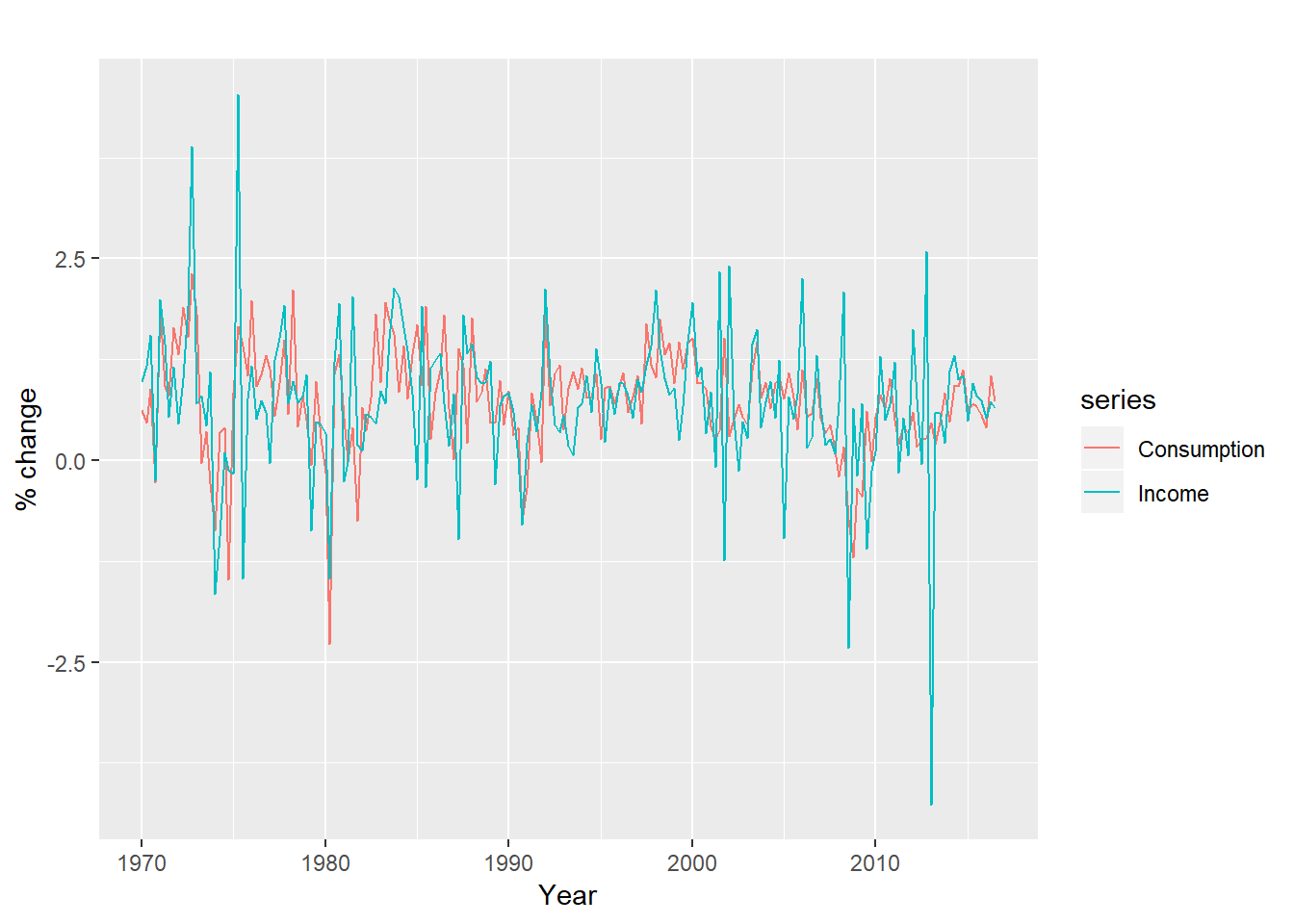Lets see the scatter plot and the regression line:

``````uschange %>%
as.data.frame() %>%
ggplot(aes(x=Income, y=Consumption)) +
ylab("Consumption (quarterly % change)") +
xlab("Income (quarterly % change)") +
geom_point() +
geom_smooth(method="lm", se=FALSE)``````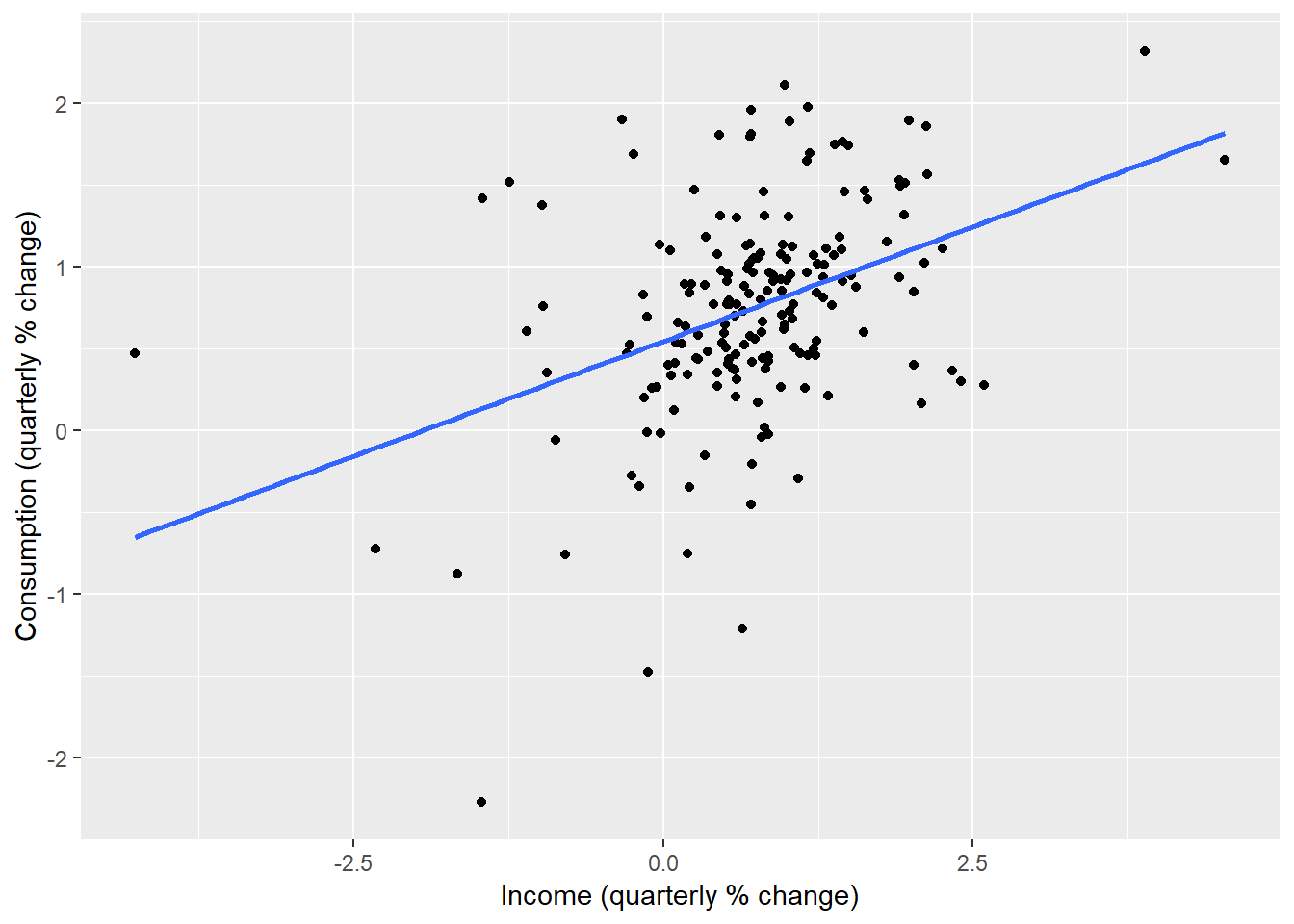We can estimate this equation using tslm() function

``tslm(Consumption ~ Income, data=uschange)``
``````##
## Call:
## tslm(formula = Consumption ~ Income, data = uschange)
##
## Coefficients:
## (Intercept)       Income
##      0.5451       0.2806``````

So every 1% increase in income consumption is increased by 0.54+0.28= 0.82%

## Multiple Linear Regression

Let us say consumption also depends upon production, saving and unemployment. Lets create a scatterplot matrix of these five variables:

``````uschange %>%
as.data.frame() %>%
GGally::ggpairs()``````
``````## Registered S3 method overwritten by 'GGally':
##   method from
##   +.gg   ggplot2``````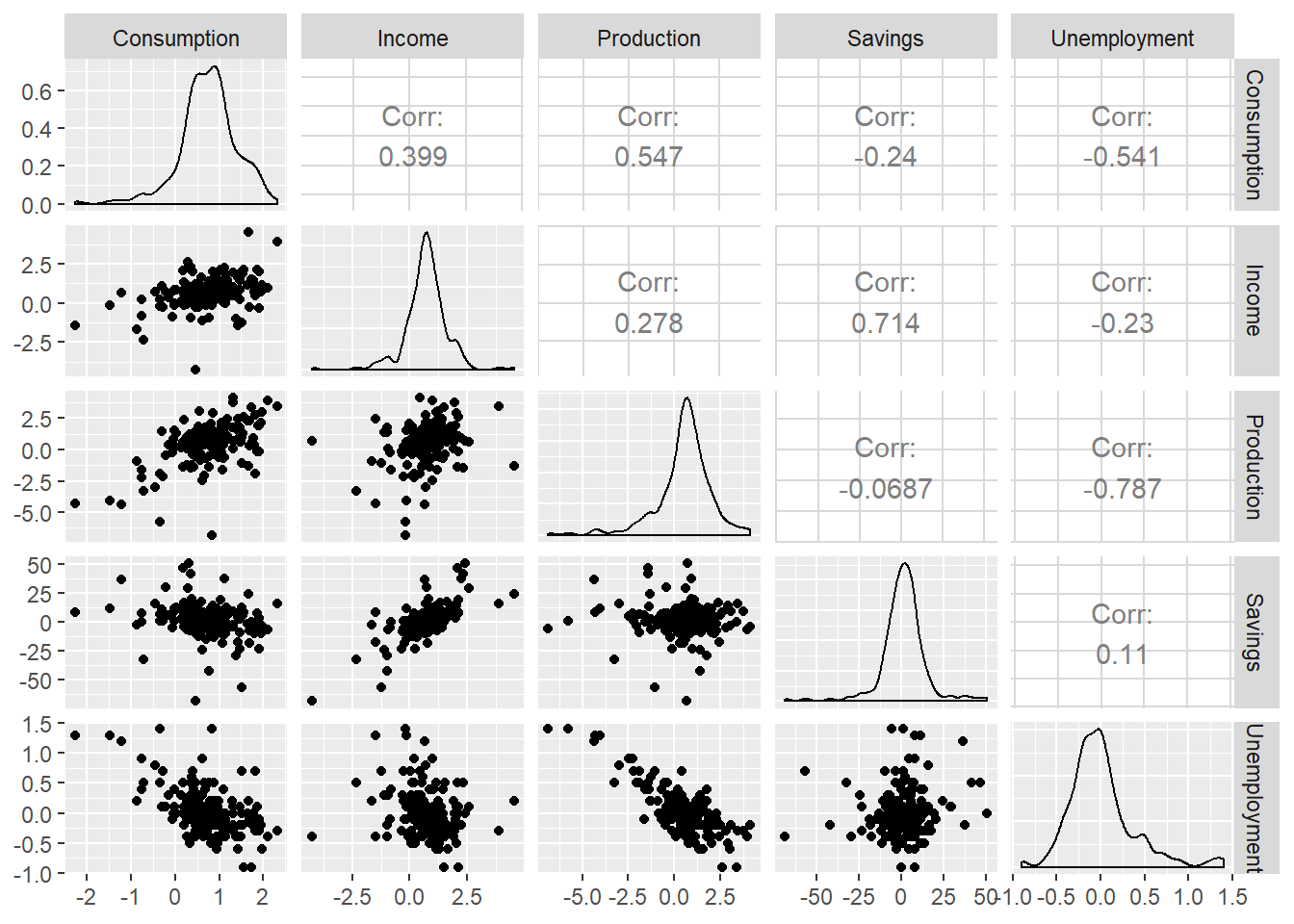tslm() function fits a linear regression model to time series data. Lets fit this model

``````fit.consMR <- tslm(
Consumption ~ Income + Production + Unemployment + Savings,
data=uschange)
summary(fit.consMR)``````
``````##
## Call:
## tslm(formula = Consumption ~ Income + Production + Unemployment +
##     Savings, data = uschange)
##
## Residuals:
##      Min       1Q   Median       3Q      Max
## -0.88296 -0.17638 -0.03679  0.15251  1.20553
##
## Coefficients:
##              Estimate Std. Error t value Pr(>|t|)
## (Intercept)   0.26729    0.03721   7.184 1.68e-11 ***
## Income        0.71449    0.04219  16.934  < 2e-16 ***
## Production    0.04589    0.02588   1.773   0.0778 .
## Unemployment -0.20477    0.10550  -1.941   0.0538 .
## Savings      -0.04527    0.00278 -16.287  < 2e-16 ***
## ---
## Signif. codes:  0 '***' 0.001 '**' 0.01 '*' 0.05 '.' 0.1 ' ' 1
##
## Residual standard error: 0.3286 on 182 degrees of freedom
## Multiple R-squared:  0.754,  Adjusted R-squared:  0.7486
## F-statistic: 139.5 on 4 and 182 DF,  p-value: < 2.2e-16``````

The value of 75.2% of R-sq suggests that the model can explain 75.4% of the variations. Lets overlap the fitted value over the actual change and see visually the similarity

``````autoplot(uschange[,'Consumption'], series="Data") +
autolayer(fitted(fit.consMR), series="Fitted") +
xlab("Year") + ylab("") +
ggtitle("Percent change in US consumption expenditure") +
guides(colour=guide_legend(title=" "))``````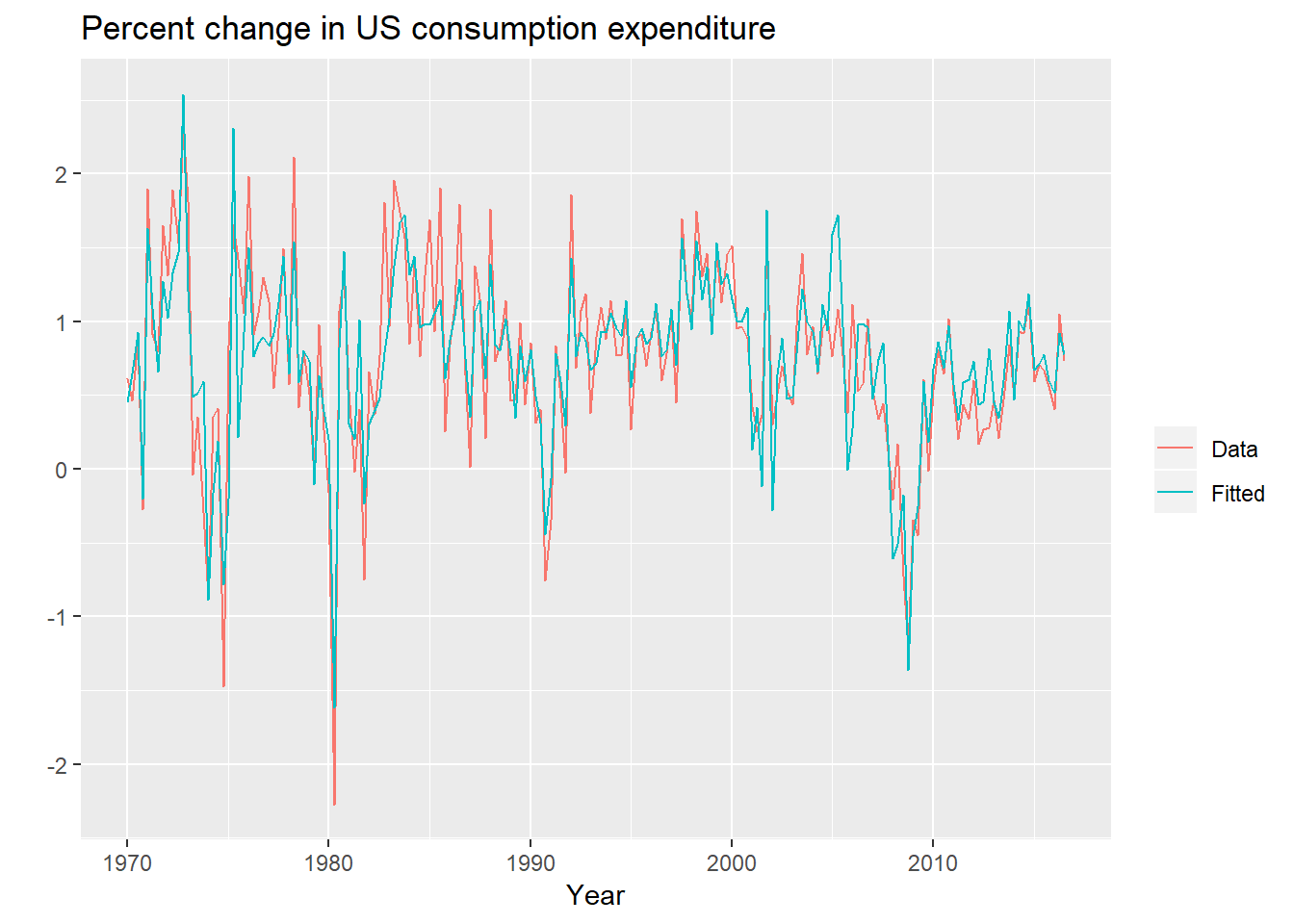lets see the scatterplot between between actual and fitted

``````cbind(Data = uschange[,"Consumption"],
Fitted = fitted(fit.consMR)) %>%
as.data.frame() %>%
ggplot(aes(x=Data, y=Fitted)) +
geom_point() +
xlab("Fitted (predicted values)") +
ylab("Data (actual values)") +
ggtitle("Percent change in US consumption expenditure") +
geom_abline(intercept=0, slope=1)``````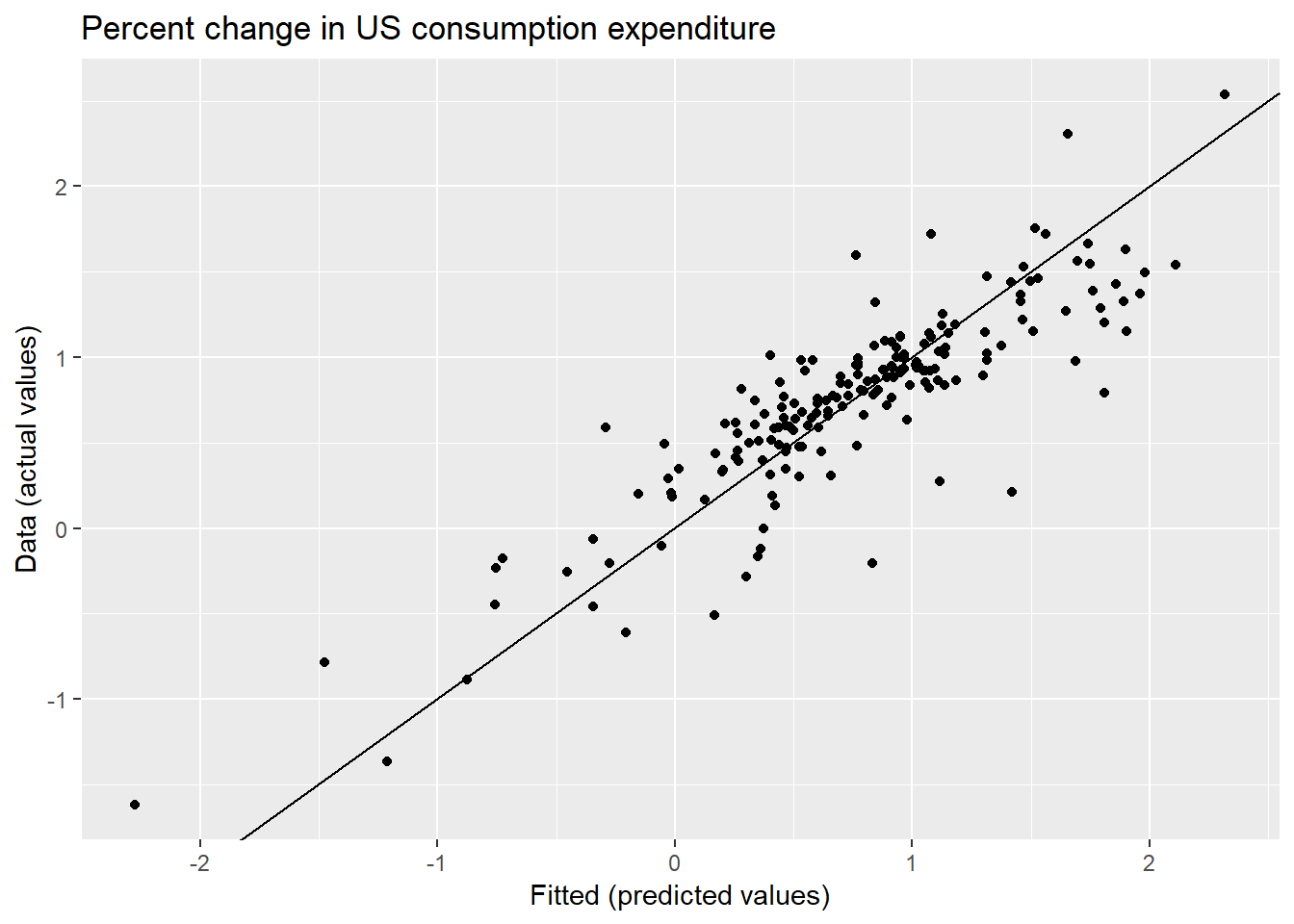## Evaluating the Regression Model

We can check the residuals

``checkresiduals(fit.consMR)``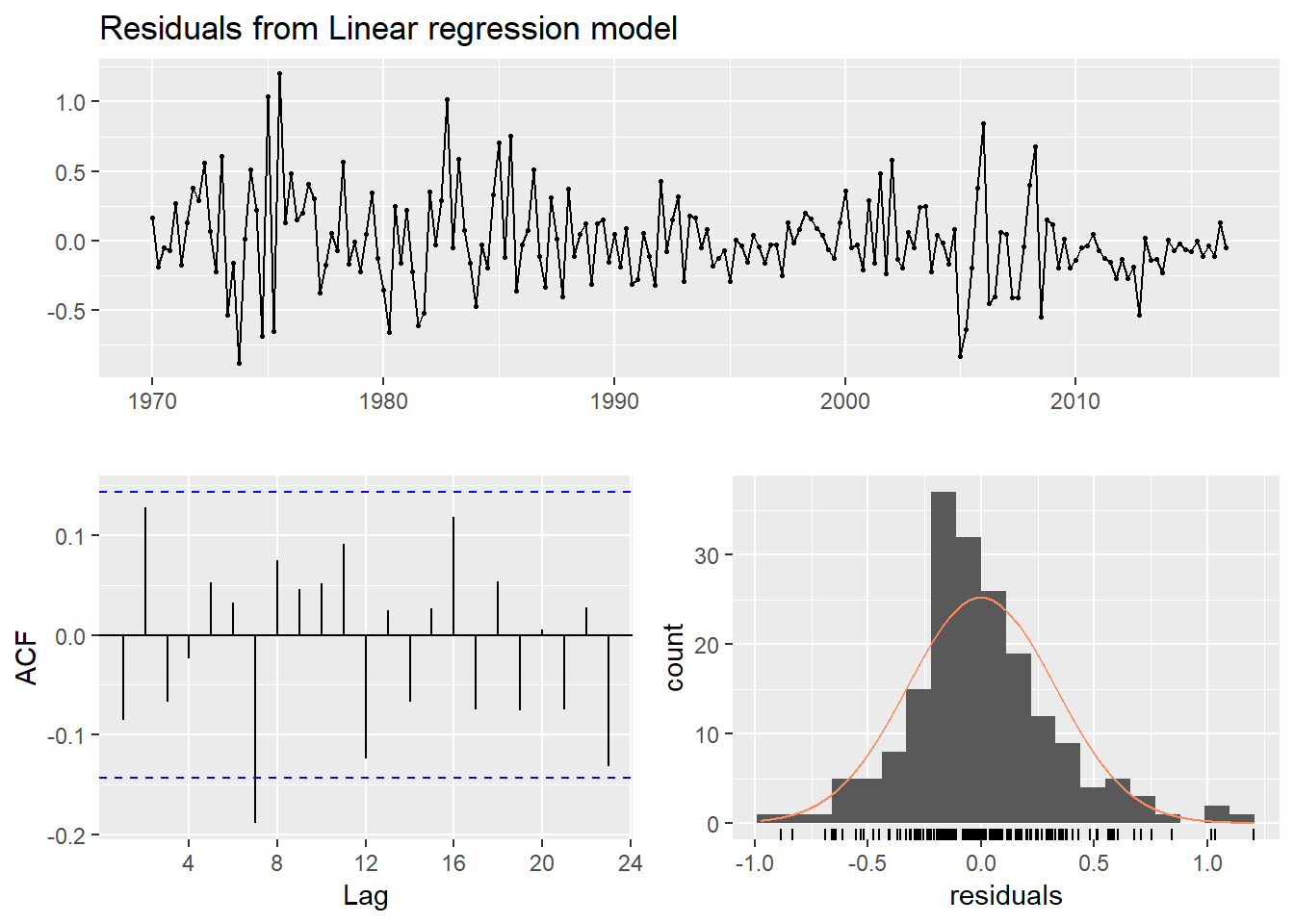``````##
##  Breusch-Godfrey test for serial correlation of order up to 8
##
## data:  Residuals from Linear regression model
## LM test = 14.874, df = 8, p-value = 0.06163``````

Timeplot: shows some changing variation over time, otherwise it is quite unremarkable Autocorrelation plot along with LM test: No autocorrelation Histogram: Slightly skewed- affect the prediction interval probability Residual Plots against predictors: Examine the scatter plot of the residuals against each of the predictor variables, if any pattern then the relationship is non linear.Modify the model Also scatter plot of the residuals against predictors not in the model, if there is a pattern, introduce them. Residual Plot against the fitted Value: if a pattern there may be a heteroscedasticity in the errors means the variance of the residuals may not be constant. Transformation needed

``````df <- as.data.frame(uschange)
df[,"Residuals"] <- as.numeric(residuals(fit.consMR))
p1 <- ggplot(df, aes(x=Income, y=Residuals)) +
geom_point()
p2 <- ggplot(df, aes(x=Production, y=Residuals)) +
geom_point()
p3 <- ggplot(df, aes(x=Savings, y=Residuals)) +
geom_point()
p4 <- ggplot(df, aes(x=Unemployment, y=Residuals)) +
geom_point()
gridExtra::grid.arrange(p1, p2, p3, p4, nrow=2)``````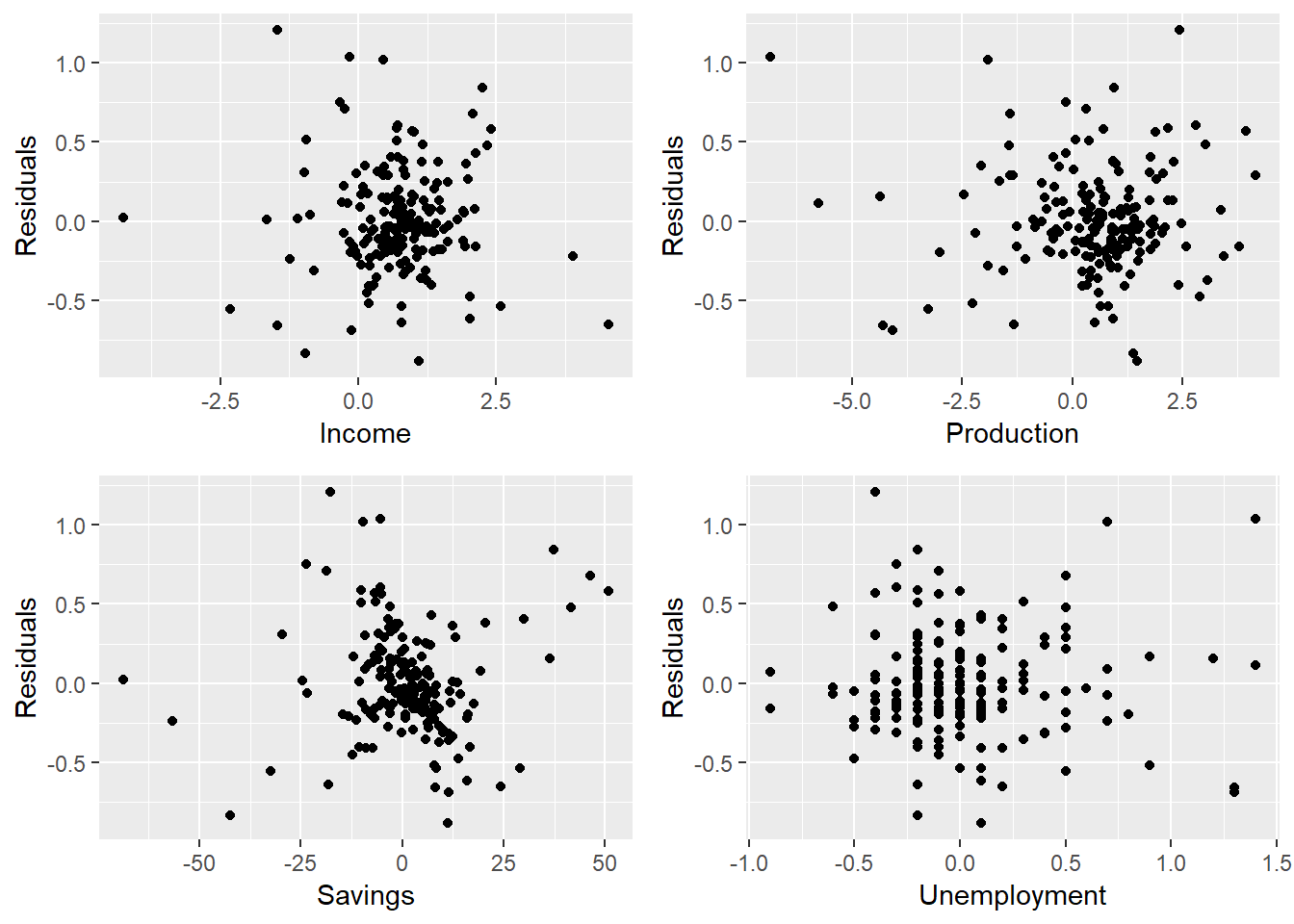Residual Plot against the fitted Value: if a pattern there may be a heteroscedasticity in the errors means the variance of the residuals may not be constant. Transformation needed

``````cbind(Fitted = fitted(fit.consMR),
Residuals=residuals(fit.consMR)) %>%
as.data.frame() %>%
ggplot(aes(x=Fitted, y=Residuals)) + geom_point()``````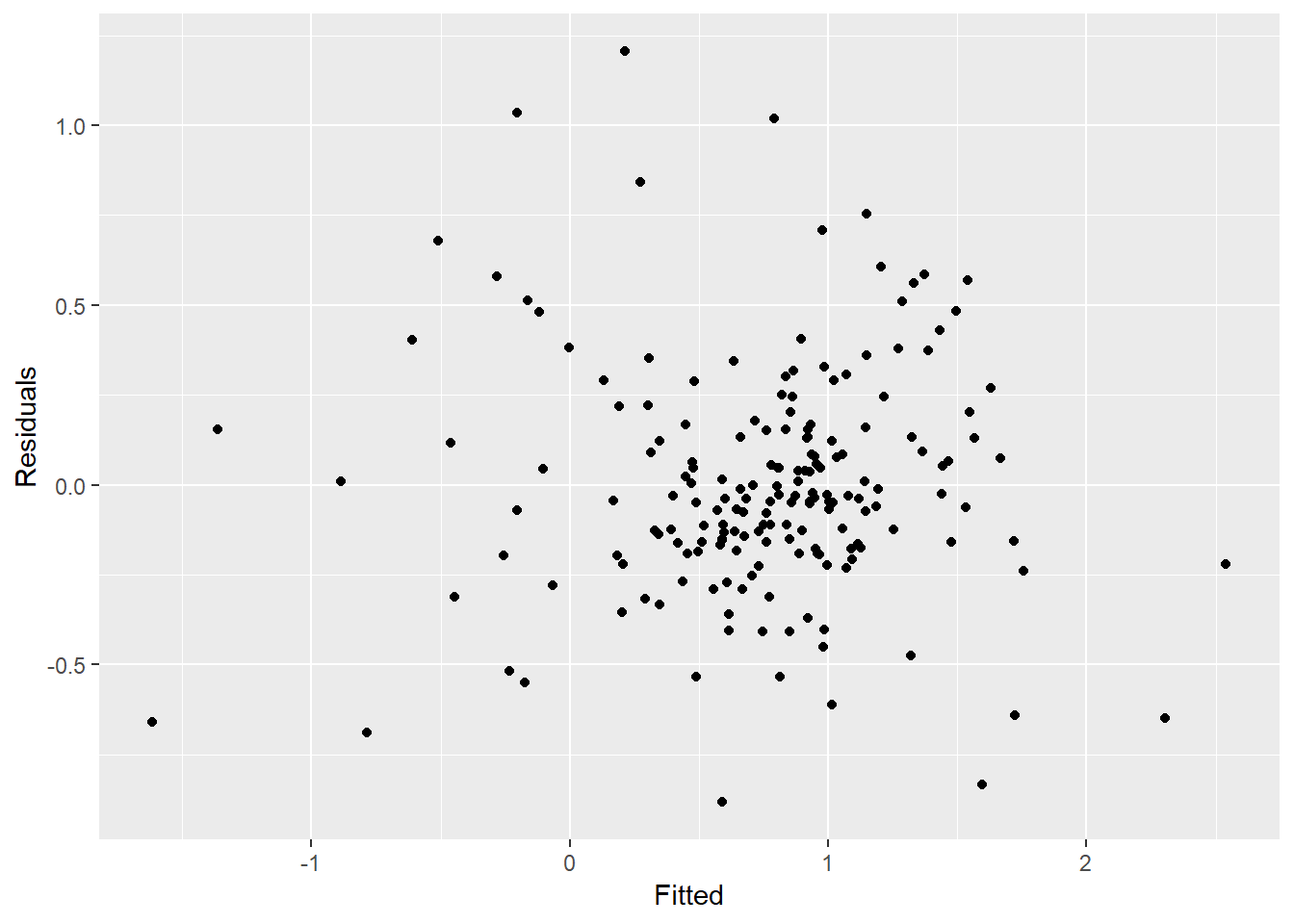There is no pattern.

Some Useful Variables 1. Trend: Can be specified in tslm() function as trend 2. Dummy Variables: tslm() autometically handles 3. Seasonal Dummy Variable: Can be specified in tslm() function as season

Australian Beer Data

``````beer2 <- window(ausbeer, start=1992)
autoplot(beer2) + xlab("Year") + ylab("Megalitres")``````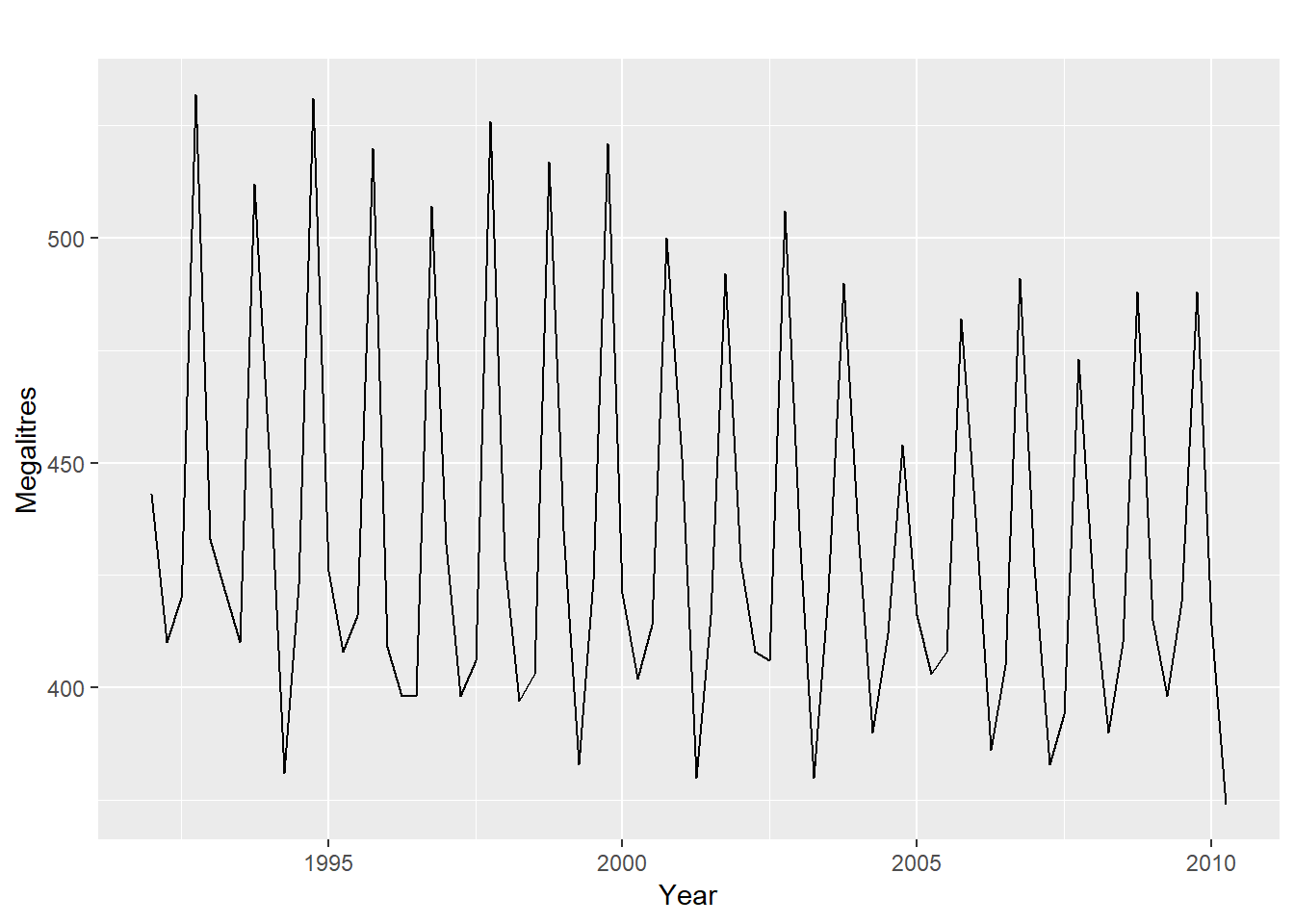We want to forecast the value of beer using a regression model with a linear trend and quarterly dummy variables

``````fit.beer <- tslm(beer2 ~ trend + season)
summary(fit.beer)``````
``````##
## Call:
## tslm(formula = beer2 ~ trend + season)
##
## Residuals:
##     Min      1Q  Median      3Q     Max
## -42.903  -7.599  -0.459   7.991  21.789
##
## Coefficients:
##              Estimate Std. Error t value Pr(>|t|)
## (Intercept) 441.80044    3.73353 118.333  < 2e-16 ***
## trend        -0.34027    0.06657  -5.111 2.73e-06 ***
## season2     -34.65973    3.96832  -8.734 9.10e-13 ***
## season3     -17.82164    4.02249  -4.430 3.45e-05 ***
## season4      72.79641    4.02305  18.095  < 2e-16 ***
## ---
## Signif. codes:  0 '***' 0.001 '**' 0.01 '*' 0.05 '.' 0.1 ' ' 1
##
## Residual standard error: 12.23 on 69 degrees of freedom
## Multiple R-squared:  0.9243, Adjusted R-squared:  0.9199
## F-statistic: 210.7 on 4 and 69 DF,  p-value: < 2.2e-16``````

Note that trend and season are created automatically by tslm. Let us visually see the fit

``````autoplot(beer2, series="Data") +
autolayer(fitted(fit.beer), series="Fitted") +
xlab("Year") + ylab("Megalitres") +
ggtitle("Quarterly Beer Production")``````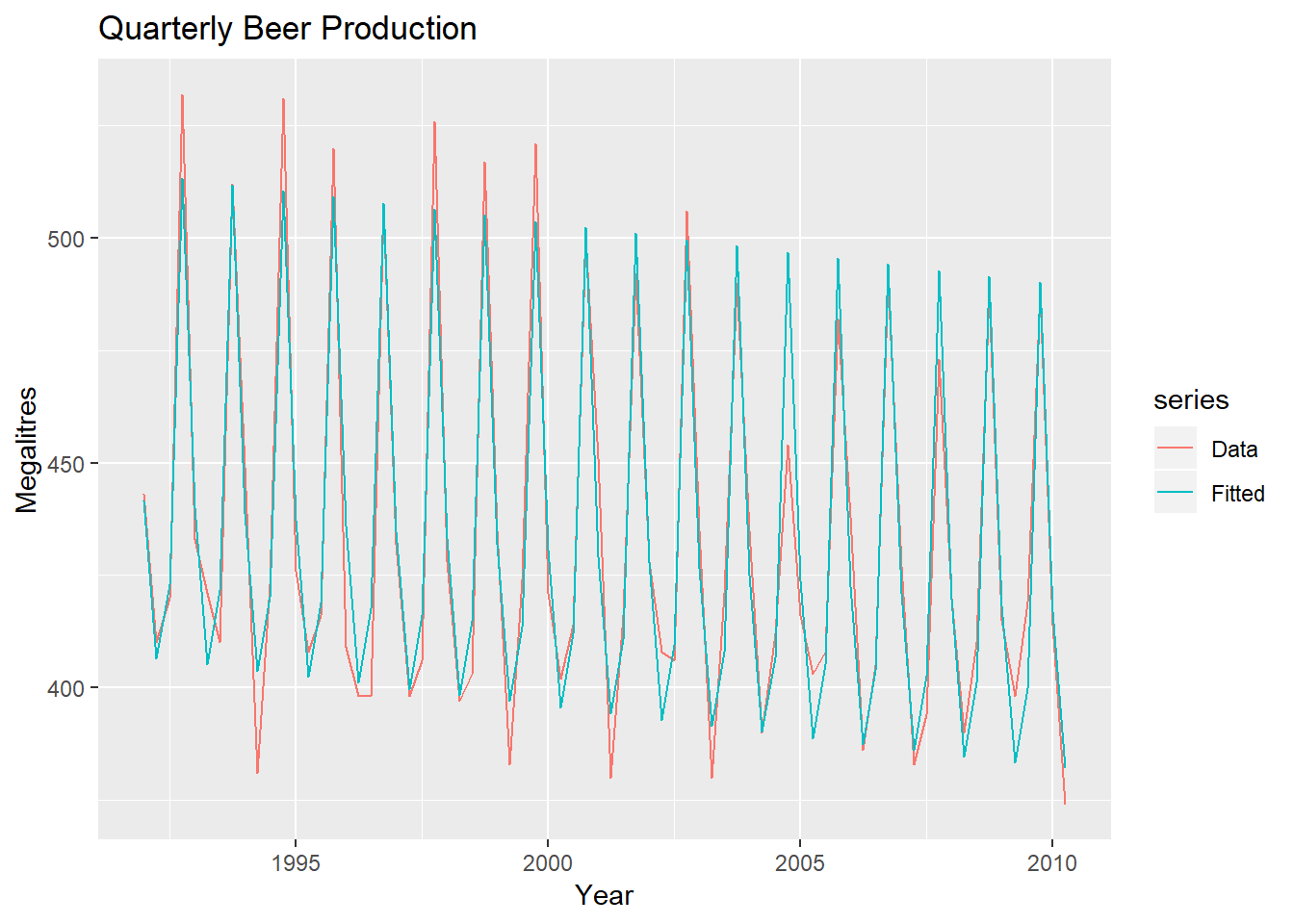Let us see the scatter plot of actual and fitted values:

``````cbind(Data=beer2, Fitted=fitted(fit.beer)) %>%
as.data.frame() %>%
ggplot(aes(x = Data, y = Fitted,
colour = as.factor(cycle(beer2)))) +
geom_point() +
ylab("Fitted") + xlab("Actual values") +
ggtitle("Quarterly beer production") +
scale_colour_brewer(palette="Dark2", name="Quarter") +
geom_abline(intercept=0, slope=1)``````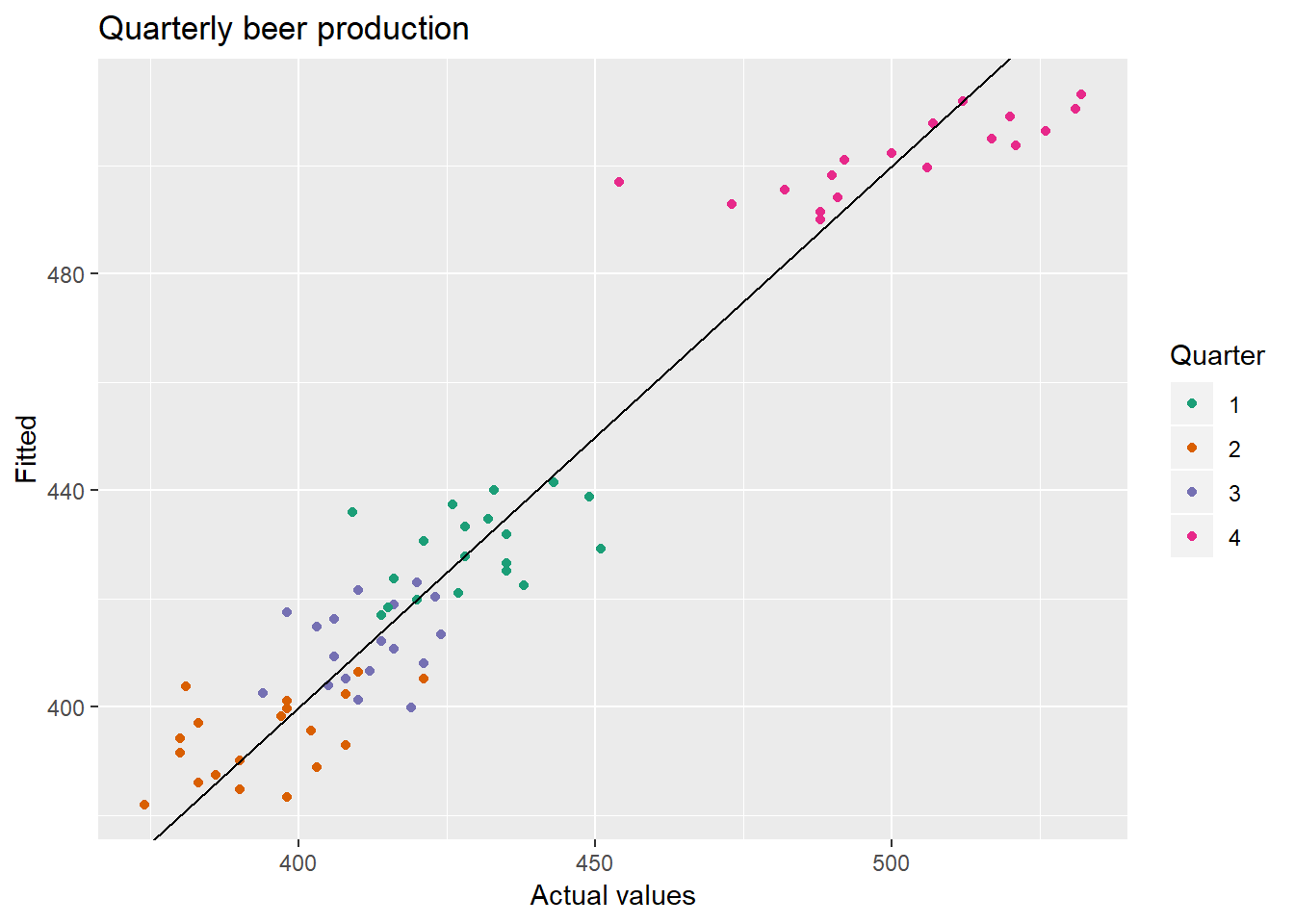###Intervention Variables

When the effect lasts only for one period, we use a “spike” variable. this is a dummy variable that takes value one in the period of the intervention and zero else where.

If an intervention causes a level shift , the value changes permanently after introduction, then we use a step variable. It takes a value 0 before intervention and 1 after.

if there is a change in slope we are talking about nonlinear trend, we discuss it later.

For monthly or quarterly data, the bizdays() will compute the number of trading days each period.

### Distributed lags

Effect of advertising lasts beyond the current period. Out of Syllabus.

## Selecting Predictors

Don’do

1. plot forecast varible against predictor- if no relationship drop that
2. Do an MLR, drop variables whose p values are >0.95

You should do

Use CV function

``CV(fit.consMR)``
``````##           CV          AIC         AICc          BIC        AdjR2
##    0.1163477 -409.2980298 -408.8313631 -389.9113781    0.7485856``````

Compare this with other models, take the value with lowest of first four and highest of last. eg. if there are 4 predictors,2^4= 16 models are possible.

Use Stepwise Regression - Forward,backward or hybrid

## Forecasting with Regression

eg beer forecast using trend & Season alone

``````beer2 <- window(ausbeer, start=1992)
fit.beer <- tslm(beer2 ~ trend + season)
fcast <- forecast(fit.beer)
autoplot(fcast) +
ggtitle("Forecasts of beer production using regression") +
xlab("Year") + ylab("megalitres")``````